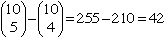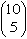Hi, I'm Edwin, a student, the question is of the second level (I guess). My problem is the following: I'm asked to come with, and program (in Ansi -C) an algorithm that calculates all the possible results of a calculation with 6 numbers and one result. For example: I want all calculations with the numbers 3, 3, 8, 8, 2, 9, and with a result of 786. all numbers may be used once, arithmetical operations allowed are + - / *, fractions are not allowed. The problem here is what is a fast method to do this (i.e. what's algorithm that can to this). I hope you can help me with this, but will not be surprised if you can't. Thank you for your time, Edwin (Netherlands). Hi Edwin, One such combination is ((9-3)*(((8*8)*2)+3)) = 786. Now if you want to find all combinations, your program has to put in the operation signs in all possible ways, that is, fill in all ? by one of the operations +, -, * or / in the following: ((9?3)?(((8?8)?2)?3)) This would be a quintuple nested statement of the type "For oi = 1 to 4, put in operation oi in spot i" (I don't know the correct syntax). This would give 45 = 1024 possible outcomes. This would have to be nested in a routine that lists the numbers 3, 3, 8, 8, 2, 9 in all possible ways in the skeleton ((blank?blank)?(((blank?blank)?blank)?blank)). The best is to view this as ((a?b)?(((c?d)?e)?f)) and ignore the fact that there are repeated entries to use the standard shuttle algorithm to list all possible permutations of a, b, c, d, e, f. This gives 6! = 720 outcomes. All of the above would have to be nested in a routine that lists all possible ways to put the parentheses consistently around a product. The one above corresponds to ((ab)(((cd)e)f)) And in all there aresuch "consistent" parenthesizing of a product. To see the correspondence, remove the last variable f and all the closings ). ((ab)(((cd)e)f)) becomes ((ab(((cde Now you build an array of 5 + and 5 - as follows: if the i-th character in the sequence is a (, then you put a +, and if it is a variable, then you put a -. ((ab(((cde becomes ++--+++--- In the sequence you obtain, the initial subsequences +, ++, ++-, ++--, ++--+, ... have the property that the number of - never exceeds the number of +. Conversely, any array of five + and five - corresponds to a consistent parenthesising of a product: ++-+--++-- becomes ((a(bc((de add f: ((a(bc((def close parentheses consistently: ((a(bc))((de)f)). This correspondence is behind the definition of Catalan numbers which you can find in most combinatorics books. However a sequence such as ++-+---++- does not correspond to a consistent parenthesizing, because the seventh initial subsequence has more - than +. You can list thearrays of five + and five - using the standard lexicographic ordering. You will dismiss most of them because they do not correspond to a parenthesizing, but on the 42 that do, you will need to form the corresponding parenthesizing, and go through the subroutine above. This will give you the 42*720*1024 = 30965760 possible outcomes. Claude Go to Math Central